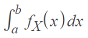# Probability and Statistics Topics IndexIf you’re looking for help with probability and statistics topics, you’re in the right place. StatisticsHowTo.com has a comprehensive database of articles covering all the material you’re likely to find in an AP statistics, elementary statistics or college statistics class.

Over a thousand articles are on the side and hundreds of those include short, how-to videos that you can also find on our YouTube Channel.
Need help with a specific homework or test question? Check out our statistics tutoring page.

## What is Probability and Statistics?

“Probability and Statistics” usually refers to an introductory course in probability and statistics. The “probability” part of the class includes calculating probabilities for events happening.

While it’s usual for the class to include basic statistics topics such as playing cards and dice rolling at first, these basic tools are used later in the class to find more complex probabilities, like the probability of contracting a certain disease using Bayes’ theorem.

The “statistics” part of probability and statistics topics includes a wide variety of methods to find actual statistics, which are numbers you can use to generalize about a population. For example, you could calculate the height of all your male classmates and find the mean height to be 5’9″; That is a statistic. But then you could take that statistic and say “I think the average height of an American male is 5’9″. How accurate your guess is depends on many factors, including how many men you measured and how many men are in the entire population. Statistics are useful because we often don’t have the resources to measure, survey or poll every member of a population, so instead we take a sample (a small amount).

We also cover the basics for calculus-based statistics. As an example, you’ll need to know definite integrals to evaluate probabilities for some random variables (X):

The probability that a ≤ X ≤ b is:Where fX is the pdf of X.

CITE THIS AS:
Stephanie Glen. "Probability and Statistics Topics Index" From StatisticsHowTo.com: Elementary Statistics for the rest of us! https://www.statisticshowto.com/probability-and-statistics/